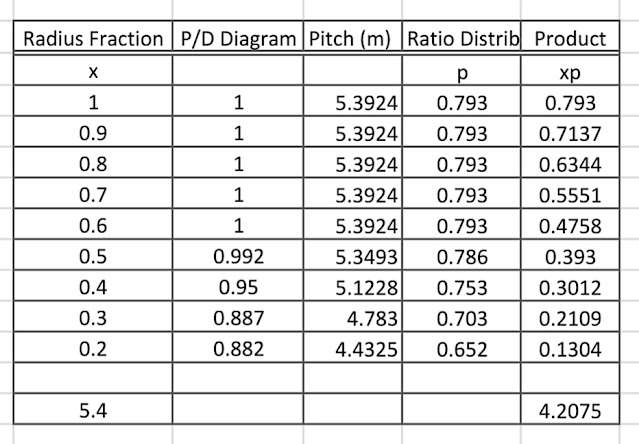## Monday, June 22, 2009

### Propeller Mean Pitch Calculation

In my previous article, I said that the pitch of propeller is determined from power coefficient Bp whose parameters are the RPM of the propelling machinery of the ship, the delivered power of the main engine, and the speed of advance. In this case, the speed of advance is the speed of water flowing toward the propeller. Also I wrote that the pitch ratio of propeller is obtained from the Bp- delta diagram. We must understand that the value which we read from the diagram is the pitch ratio at the tip of the propeller.
If we use B-series propeller as our standard for the design of our propeller, then we must calculate the mean pitch ratio of the designed propeller. The pitch of B-series or Troost propeller varies according to its radius. It means the surface of the propeller is spiral. If the generatrix or generator line of a propeller has curved form then the pitch distribution of the propeller is not linear.
The following table is an example of mean pitch ratio calculation for an Open Hatch Bulk Carrier of 45,000 DWT. Based on the data of the B-series provided by T.P. O'Brien in his book, The Design of Marine Screw Propeller on page 132. The tip pitch ratio is 0.793 and the diameter of the propeller is 6.8 meters.Propeller Mean Pitch Calculation
After tabulating the calculation of the pitch distribution based on P/D diagram, the product of pitch and radius x will have to be devided with the sum of the radius fraction of the propeller. Mean Pitch Ratio: sum of xp/  sum of x =4.2075/ 5.4 = 0.779.
Propeller is still the most common form of propulsion device that propells ships around the world. By studying the propulsion properties of propellers we can design high efficient propellers that can move ships at sea in higher speed, with much less vibration and low fuel oil consumption.So, the mean pitch ratio (P/D)mean of the designed propeller is 0.779
by Charles Roring in Manokwari of West Papua - Indonesia